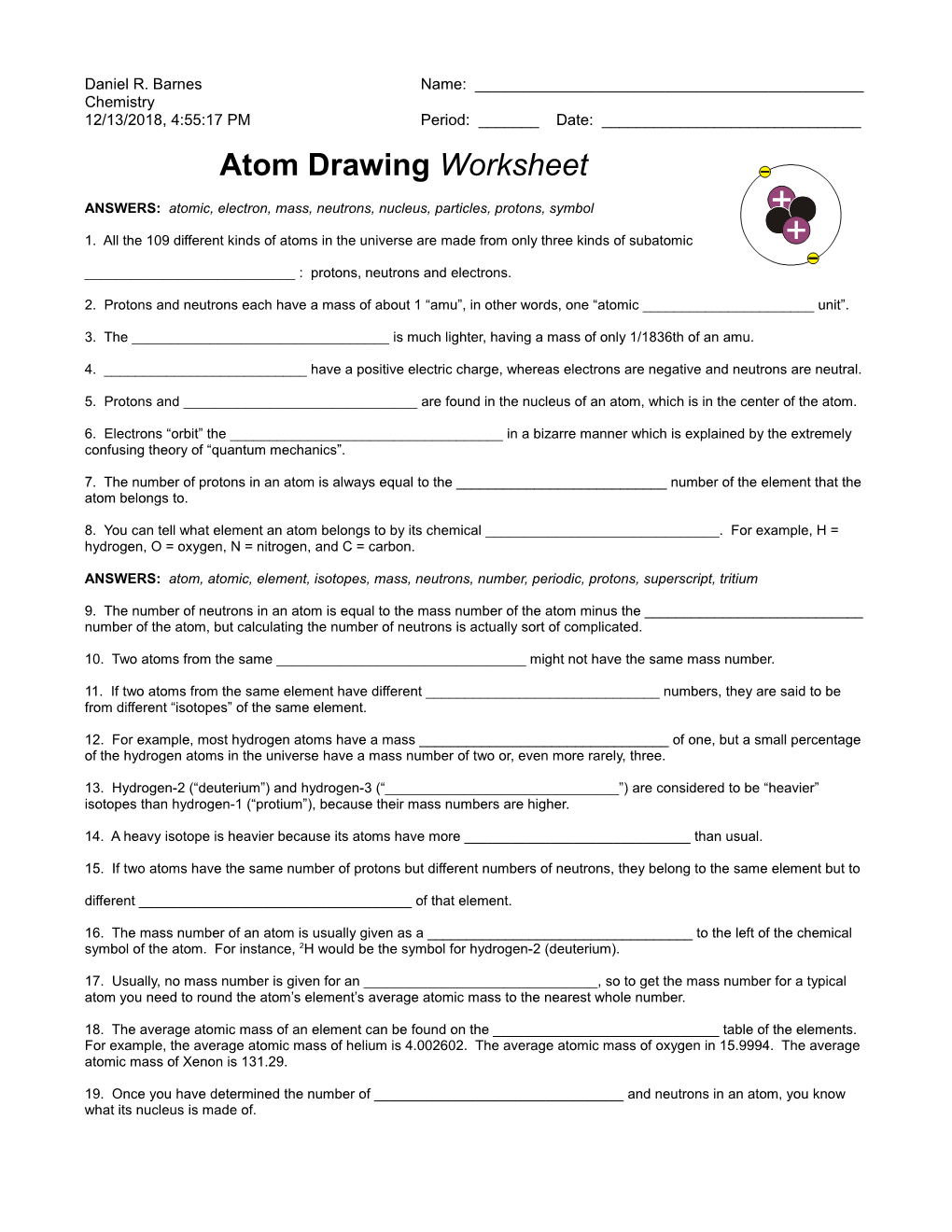# Atom Drawing Worksheet, Daniel R. Barnes, Chemistry, 9/9/05 10:08:25 AMAtom Drawing Worksheet, Daniel R. Barnes, Chemistry, 9/9/05 10:08:25 AM

Daniel R. BarnesName: ______

Chemistry

12/25/2018, 7:20:25 AMPeriod: ______Date: ______

Atom Drawing Worksheet

ANSWERS: atomic, electron, mass, neutrons, nucleus, particles, protons, symbol

1. All the 109 different kinds of atoms in the universe are made from only three kinds of subatomic

______: protons, neutrons and electrons.

2. Protons and neutrons each have a mass of about 1 “amu”, in other words, one “atomic ______unit”.

3. The ______is much lighter, having a mass of only 1/1836th of an amu.

4. ______have a positive electric charge, whereas electrons are negative and neutrons are neutral.

5. Protons and ______are found in the nucleus of an atom, which is in the center of the atom.

6. Electrons “orbit” the ______in a bizarre manner which is explained by the extremely confusing theory of “quantum mechanics”.

7. The number of protons in an atom is always equal to the ______number of the element that the atom belongs to.

8. You can tell what element an atom belongs to by its chemical ______. For example, H = hydrogen, O = oxygen, N = nitrogen, and C = carbon.

ANSWERS: atom, atomic, element, isotopes, mass, neutrons, number, periodic, protons, superscript, tritium

9. The number of neutrons in an atom is equal to the mass number of the atom minus the ______number of the atom, but calculating the number of neutrons is actually sort of complicated.

10. Two atoms from the same ______might not have the same mass number.

11. If two atoms from the same element have different ______numbers, they are said to be from different “isotopes” of the same element.

12. For example, most hydrogen atoms have a mass ______of one, but a small percentage of the hydrogen atoms in the universe have a mass number of two or, even more rarely, three.

13. Hydrogen-2 (“deuterium”) and hydrogen-3 (“______”) are considered to be “heavier” isotopes than hydrogen-1 (“protium”), because their mass numbers are higher.

14. A heavy isotope is heavier because its atoms have more ______than usual.

15. If two atoms have the same number of protons but different numbers of neutrons, they belong to the same element but to

different ______of that element.

16. The mass number of an atom is usually given as a ______to the left of the chemical symbol of the atom. For instance,2H would be the symbol for hydrogen-2 (deuterium).

17. Usually, no mass number is given for an ______, so to get the mass number for a typical atom you need to round the atom’s element’s average atomic mass to the nearest whole number.

18. The average atomic mass of an element can be found on the ______table of the elements. For example, the average atomic mass of helium is 4.002602. The average atomic mass of oxygen in 15.9994. The average atomic mass of Xenon is 131.29.

19. Once you have determined the number of ______and neutrons in an atom, you know what its nucleus is made of.

ANSWERS: atom, atomic, charge, electric, electrons, extra, ion, missing, negatively, neutral, positively, protons, superscript, unequal

20. In order to know how many ______are in an atom, you need to know the atom’s overall electric charge.

21. The electric charge of an atom is written as a ______to the right of the atom’s chemical symbol.

22. If an ______is neutral, there will be no charge shown.

23. If an atom is ______, the number of electrons will equal the number of protons.

24. In other words, if an atom is neutral, the number of electrons will equal the ______number of the atom’s element.

25. However, many atoms in the universe have ______numbers of protons and electrons.

26. If the number of ______in an atom does not equal the number of electrons in the atom, the atom is considered to be an “ion”.

27. If an atom has more electrons than usual, it will be a ______-charged “anion”.

28. If an atom is missing electrons, it will be a ______-charged “cation”.

29. The superscript in the upper right of an ion’s symbol will tell you the ______charge of the ion, which can tell you how many extra electrons it has or how many electrons it is missing.

30. For example, Li+ is a lithium ion with one ______electron.

31. O2- is an oxygen ion with two ______electrons. N3- is a nitrogen ion with three extra electrons. Be2+ is a beryllium ion with two electrons missing.

32. Remember that a negative electric charge indicates that the ______has extra electrons.

33. Remember that a positive electric ______means that an ion is missing electrons.

ASSIGNMENT: Draw twelve or more of the following atoms on one or more separate sheets of paper, using the method taught to you by your instructor. WARNING: One of the following is a trick question, an atom that can not exist. Indicate which one. Instead of drawing it, explain why it can’t be drawn.

LiLi+C14COO2-18O18O2-HeH2H3HBe2+Ne

H+3H-BeH3-He2+B6B3+N13+N3-FF-Mg30S2-Ar

Some things to remember as you draw your atoms:

Show your math with the same level of detail that your instructor shows when he does sample problems.

Make sure to show why you’re using the numbers you are. Arrows are handy for this.

Give each atom some elbow room. Don’t let an atom get too close to any of your math or too close to another atom. On the atom drawing quiz, you will be drawing only six atoms on one side of a piece of paper.

Make the symbols for protons and neutrons about the same size as each other, but make the electron’s symbol visibly smaller than the proton and the neutron. Your protons and neutrons should each be about 6 mm wide, the size of a pea. Your electrons should be about 3 mm in diameter.